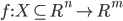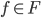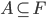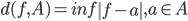Approximation Capabilities of Neural Networks. C. Enăchescu, Journal of Numerical Analysis, Industrial and Applied Mathematics (JNAIAM), vol. 3, no. 3-4, 2008, pp. 221-230

From the learning point of view, the approximation of a function is equivalent with the learning problem of a neural network. In this paper we want to show the capabilities of a neural network to approximate arbitrary continuous functions. We have made some experiments in order to confirm the theoretical results.

c48613aed54c29e0f60ca35833aebf70.pdf$f : X \subseteq R^{n} \to R^{m}$ is a continuous function

## Neural Network and Best Approximation Theory

Given$f \in F$ and$A \subseteq F$ we call the distance of f from A as$d(f,A)=inf\left \| f-a \right \|, a\in A$